## MySQL_Table_Select 原

1. Select field

``````select id, stuName, sex from t_student;
select * from t_student;``````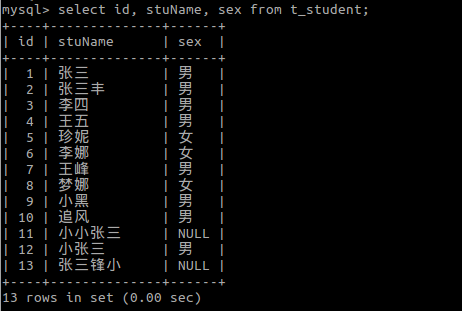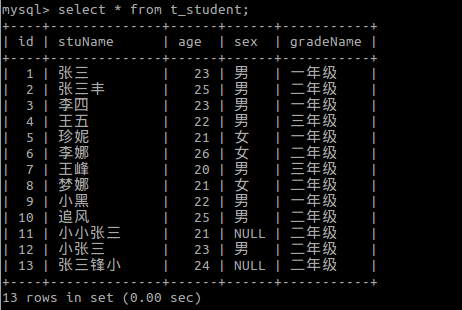2. Select + where

``````select * from t_student where id=7;
select * from t_student where age>22;``````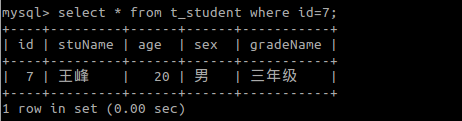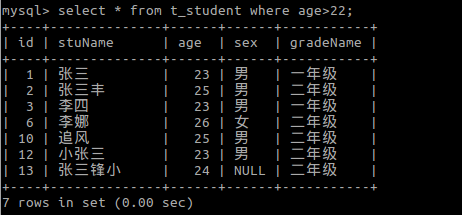3. Select + in

``````select * from t_student where age in (21, 23);
select * from t_student where age not in (21, 23);``````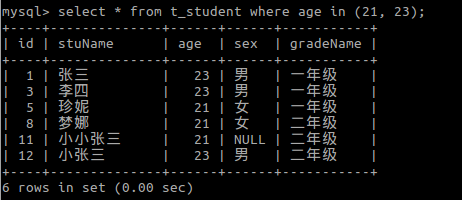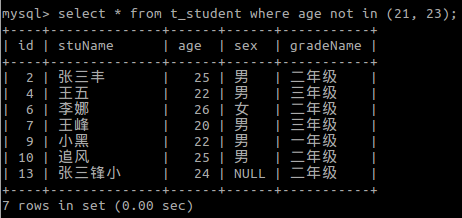4. Select + between ... and ...

``````select * from t_student where age between 21 and 24;
select * from t_student where age not between 21 and 24;``````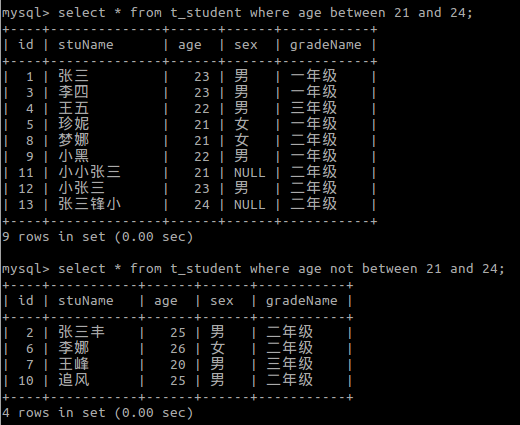5. Select + like

``````select * from t_student where stuName like '张三';
select * from t_student where stuName like '张三%';
select * from t_student where stuName like '张三_';
select * from t_student where stuName like '%张三%';``````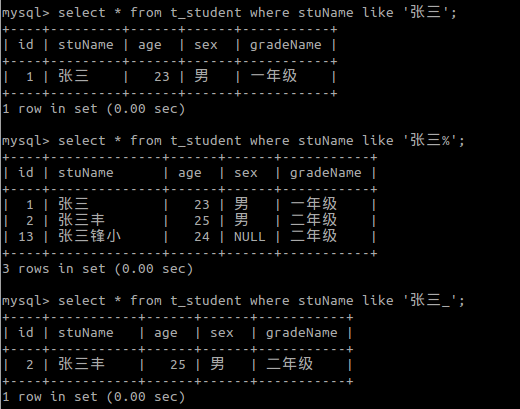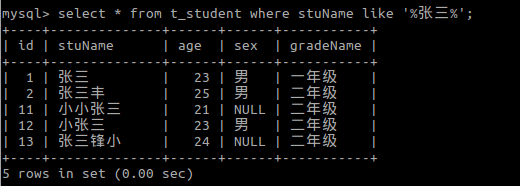ps: %: any word;

_: only one word;

6. Select + is null

``````select * from t_student where sex is null;
select * from t_student where sex is not null;``````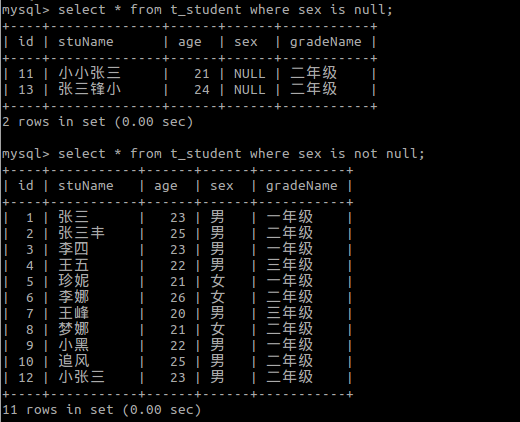7. Select + and

``select * from t_student where gradeName='一年级' and age=23;``8. Select + or

``select * from t_student where gradeName='一年级' or age=23;``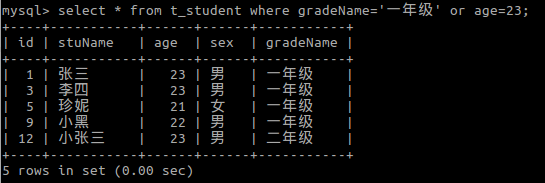9. Select + distinct

``select distinct gradeName from t_student;``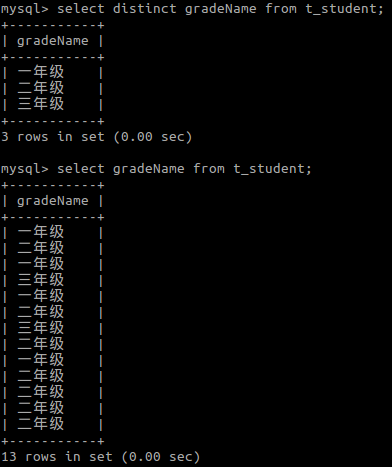10. Select + order by

``````select * from t_student order by age;
select * from t_student order by age asc;
select * from t_student order by age desc;``````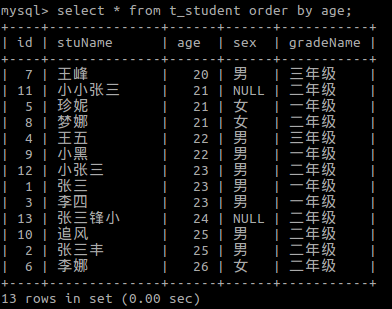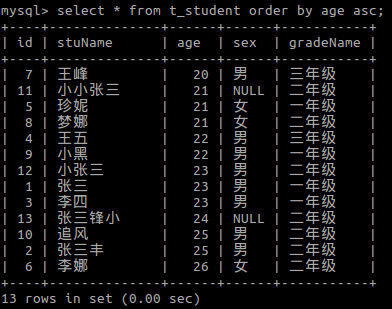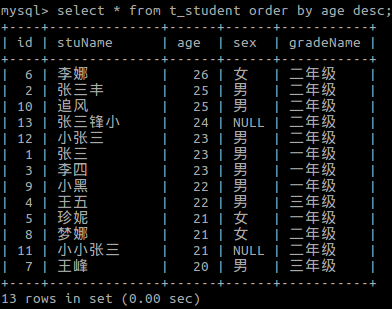11. Select + group by

``````select * from t_student group by gradeName;        /* Useless for only 'group by' */
select gradeName, group_concat(stuName) from t_student group by gradeName;
/* group_concat */
select gradeName, count(stuName) from t_student group by gradeName;
/* Aggregate function: count() */
select gradeName, count(stuName) from t_student group by gradeName having count(stuName)>3;
/* With having condition */
select gradeName, count(stuName) from t_student group by gradeName with rollup;
select gradeName, group_concat(stuName) from t_student group by gradeName with rollup;
/* With rollup *``````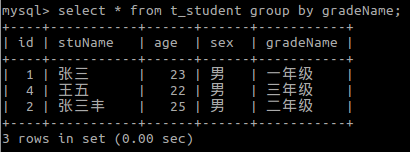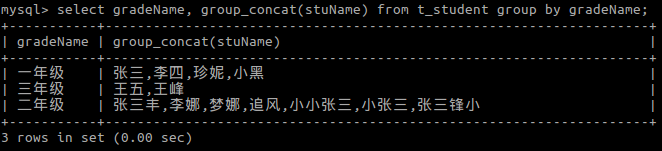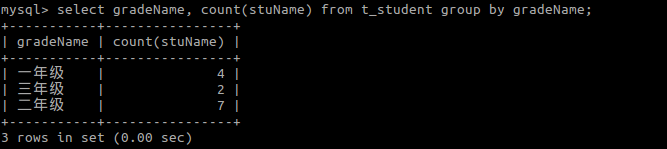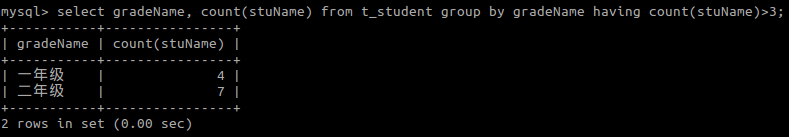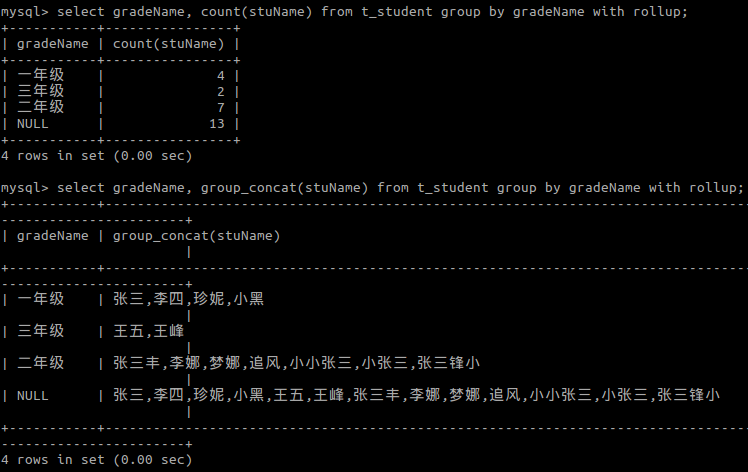12. Select + limit

``````select * from t_student limit 0, 5;
select * from t_student limit 5, 5;
select * from t_student limit 10, 5;``````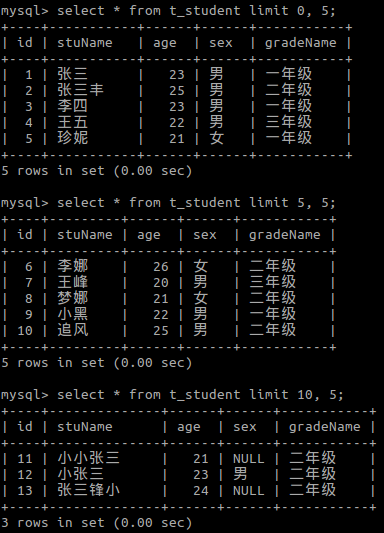### 大饼脸

2014/07/09
65
0
SQL Server中数据表、视图、函数/方法、存储过程是否存在判断及创建

Jansens
2016/11/01
81
0
MySQL数据库中如何使用rand随机查询记录

ycy蓝码
2016/03/10
0
0
MySQL运维常用的SQL语句

###################分析类############################################################### -- 查询表中数据超过1000行的表 select concat(tableschema,'.',tablename) as tablename,tabl......

2018/05/09
0
0
[我在学php之三]Po上自己写的数据库类，方便以后进行查找。

class mysql{ private \$conn; //私有化 变量\$connprivate \$dbhost; //主机private \$dbuser;private \$dbpassword;private \$dbname; //数据库名称 function construct(\$dbhost,\$dbuser,\$dbpass......

2015/05/11
102
2

34分钟前
4
0

37分钟前
6
0
springmvc jsp访问不了css

39分钟前
4
0
android 系统版本 9.1网络请求遇到坑

MrLins
56分钟前
5
0
Istio 1.3 发布，HTTP 遥测不再需要 Mixer

> 原文链接：Istio 1.3 发布，HTTP 遥测不再需要 Mixer Istio 是 Google、IBM 和 Lyft 联合开源的服务网格（Service Mesh）框架，旨在解决大量微服务的发现、连接、管理、监控以及安全等问题...

10
0